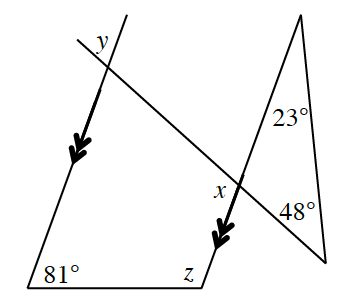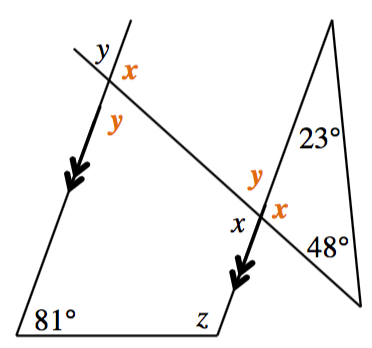### Home > INT2 > Chapter 9 > Lesson 9.1.2 > Problem9-22

9-22.Examine the diagram at right. Use the given geometric relationships to solve for $x$, $y$, and $z$. Be sure to justify your work by stating the geometric relationship and applicable theorem.

There are some other angles in the diagram that have the same measures as $x$ and $y$. Which ones?